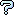All about flooble | fun stuff | Get a free chatterbox | Free JavaScript | Avatarsperplexus dot infoThe Island of Masyu (Posted on 2006-06-06)Located in the southwestern area of the Rhubarb Sea is the tropical island of Masyu, a square-shaped isle recently settled by 25 Ghruthans -- countrymen of a kingdom comprised of only Knights and Knaves.

In accordance with the decree of their King (who is a Knight and author of the Royal Ghruthan Building Code), the island was staked off into one hundred lots, with 25 of these lots each homesteaded by the King and 24 other Ghruthan settlers. And, to encourage commerce and cooperation on the island, the settlers had built a road that connected each and every settled lot within the strict guidelines imposed by their King.

The following is an excerpt of the Royal Ghruthan Island Building Code:

• XI.a. Only a single road shall ever be built on the island. The road shall be a continuous non-intersecting loop and pass through all settled lots on the island. Any road which passes through a lot shall enter through the center of one of the lot�s four sides and exit through the center of a different side of the lot.
• XI.b.1. Any road built passing through a Knight�s lot shall exit the lot on the side directly opposite the side it entered. In addition, the road shall be built so that it makes at least one right angle turn in the lot immediately preceding or following the Knight�s lot.
• XI.b.2 Any road built passing through a Knave�s lot shall turn at a right angle within the lot. In addition, the road shall not bend but travel straight through the lots immediately preceding and following the Knave�s lot.

```  1   2   3   4   5   6   7   8   9   0
+===+===+===+===+===+===+===+===+===+===+
|   | X |   | X |   |   |   | O |   |   | A
+===+===+===+===+===+===+===+===+===+===+
|   |   |   |   | O |   |   |   |   | O | B
+===+===+===+===+===+===+===+===+===+===+
|   |   |   |   |   | O |   |   |   |   | C
+===+===+===+===+===+===+===+===+===+===+
| O |   | O |   |   |   |   | X |   | O | D
+===+===+===+===+===+===+===+===+===+===+
|   |   | X |   |   |   |   |   |   | X | E
+===+===+===+===+===+===+===+===+===+===+
|   | O | O |   |   |   | O |   |   |   | F
+===+===+===+===+===+===+===+===+===+===+
| X |   |   |   | O |   |   | X | O |   | G
+===+===+===+===+===+===+===+===+===+===+
|   |   |   |   | O | O |   |   |   |   | H
+===+===+===+===+===+===+===+===+===+===+
|   |   | O |   |   |   |   |   |   | X | I
+===+===+===+===+===+===+===+===+===+===+
| X |   | X |   |   |   | O |   |   |   | J
+===+===+===+===+===+===+===+===+===+===+
```

You have been given a copy of the map of Masyu created prior to the construction of its roadway. Each settled lot was pre-marked with an �X� to indicate it was settled by a Knave, or an �O� to indicate it was settled by a Knight. From only the map, knowledge of the Royal Ghruthan Island Building Code, and the following conversation overheard between two Ghruthans, can you locate the King? If you can, identify his lot.
(Note: to a Ghruthan, a neighbor is next or prior, though not necessarily adjacent, along the road).

A: �My lot is adjacent to the Rhubarb Sea.�
B: �I settled adjacent to the sea. There is no settled lot orthogonally adjacent to my lot.�
A: �There is a settled lot diagonally adjacent to my lot.�
B: �My neighbors are either Knights or both Knaves.�
A: �The King is my neighbor. The King lives on the coast.�
B: �The King�s nearest neighbor is a Knave. The King does not live on the coast.�
A: �I have no Knave as a neighbor.�
B: �Both my neighbors have a Knave as a neighbor.�

 No Solution Yet Submitted by Dej Mar Rating: 3.0000 (3 votes)Subject Author Datere: Solution Dej Mar 2006-06-18 19:10:18Solution Eric 2006-06-06 20:28:27? : / Josh 2006-06-06 17:09:38The road (a start) Jyqm 2006-06-06 16:12:40Please log in:

 Search: Search body:
Forums (0)